Here is this month's selection of Traders' Tips, contributed by various developers of technical analysis software to help readers more easily implement some of the strategies presented in this and other issues.

You can copy these formulas and programs for easy use in your spreadsheet or analysis software. Simply "select" the desired text by highlighting as you would in any word processing program, then use your standard key command for copy or choose "copy" from the browser menu. The copied text can then be "pasted" into any open spreadsheet or other software by selecting an insertion point and executing a paste command. By toggling back and forth between an application window and the open Web page, data can be transferred with ease.

This month's tips include formulas and programs for: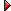TRADESTATION: DETECTING BREAKOUTS INTRADAYTRADESTATION: CHART SENTIMENT INDEXMETASTOCK: DETECTING BREAKOUTS INTRADAYMETASTOCK: CHART SENTIMENT INDEXAMIBROKER: DETECTING BREAKOUTS INTRADAYAMIBROKER: INDEX OF CHART SENTIMENTeSIGNAL: DETECTING BREAKOUTS INTRADAYWEALTH-LAB: DETECTING BREAKOUTS INTRADAYWEALTH-LAB: INDEX OF CHART SENTIMENTNEUROSHELL TRADER: DETECTING BREAKOUTS INTRADAYNEUROSHELL TRADER: INDEX OF CHART SENTIMENTNEOTICKER: DETECTING BREAKOUTS INTRADAYNEOTICKER: INDEX OF CHART SENTIMENTTRADINGSOLUTIONS: DETECTING BREAKOUTS INTRADAYINVESTOR/RT: DETECTING BREAKOUTS INTRADAYINVESTOR/RT: CHART SENTIMENT INDEXFINANCIAL DATA CALCULATOR: DETECTING BREAKOUTS INTRADAYTRADESTATION: DETECTING BREAKOUTS INTRADAY

Markos Katsanos' article in this issue, "Detecting Breakouts In Intraday Charts," describes a volatility adjustment to his finite volume element (FVE) calculation, which Katsanos first presented in the April 2003 issue of STOCKS & COMMODITIES.

Katsanos includes some TradeStation code for his indicator in a sidebar to the article. We have enhanced Katsanos' code by putting the volatility-adjusted Fve formula into an independent function, which the other routines all use. This reduces the amount of code slightly and also ensures that all indicators use the same formula. In addition, we have inserted a test for intraday bars. This makes it a bit easier to ensure that the word "ticks" is reserved for obtaining volume numbers when intraday and tick bars are used.

Function: FveFactorWVolatility

{ Volatility modified FVE formula

```Katsanos, Detecting Breakouts in Intraday Charts }
inputs:
Samples( numericsimple ),
CInter( numericsimple ),
CIntra( numericsimple ) ;
variables:
TP( 0 ),
Intra( 0 ),
VIntra( 0 ),
Inter( 0 ),
VInter( 0 ),
Cutoff( 0 ),
MF( 0 ) ;
TP = ( High + Low + Close ) / 3 ;
Intra = Log( High ) - Log( Low ) ;
Vintra = StandardDev( Intra, Samples, 1 ) ;
if TP > 0 then
Inter = Log( TP ) - Log( TP ) ;
Vinter = StandardDev( Inter, Samples, 1 ) ;
Cutoff = CIntra * VIntra + CInter * VInter ;
MF = ( Close - ( High + Low ) / 2) + TP - TP ;
if MF > CutOff * Close then
FveFactorWVolatility = 1
else if MF < -1 * CutOff * Close then
FveFactorWVolatility = -1
else FveFactorWVolatility = 0 ;```
Indicator: FVEwithVolatility
```{ Volatility modified FVE formula
Katsanos, Detecting Breakouts in Intraday Charts }
inputs:
Samples( 22 ),
PerMA( 40 ),
CIntra( .1 ),
CInter( .1 );
variables:
VolumePlusMinus( 0 ),
FVE( 0 ),
FVESum( 0 ),
MyVolume( 0 ) ;
if BarType < 2 then
MyVolume = Ticks
else
MyVolume = Volume ;
if BarNumber > Samples then
begin
VolumePlusMinus = Volume *
FveFactorWVolatility( Samples, CInter, CIntra ) ;
FVEsum = Summation( VolumePlusMinus, Samples ) ;
FVE = ( FVEsum / ( Average( Volume, Samples )
* Samples ) ) * 100 ;
Plot1( Average( FVE, 1 ), "FVE" ) ;
Plot2( XAverage( FVE, PerMA ), "EMAFVE" ) ;
Plot3( 0, "0" ) ;
if ( FVE > -20 and FVE < 10 )
and FVE > XAverage( FVE, PerMA )
and LinearRegAngleFC( FVE, 20 ) > 30
then
end ;
Indicator: FVEVolBars
{ Volatility color-coded Volume bar formula
Katsanos, Detecting Breakouts in Intraday Charts }
inputs:
AvgLength( 50 ),
UpColor( Green ),
DownColor( Red ),
NeutralColor( Blue ),
CIntra( .1 ),
CInter( .1 ),
Samples( 22 ) ;
variables:
MyVolume( 0 ),
MyFVEFactor( 0 ) ;
MyFVEFactor = FveFactorWVolatility( Samples, CInter, CIntra ) ;
if BarType < 2 then
MyVolume = Ticks
else
MyVolume = Volume ;
Plot1( MyVolume, "Vol" ) ;
Plot2( AverageFC( MyVolume, AvgLength ), "VolAvg" ) ;
{ Color criteria }
If MyFVEFactor = 1  then
SetPlotColor( 1, UpColor )
Else if MyFVEFactor = -1 then
SetPlotColor( 1, DownColor )
else SetPlotColor( 1, NeutralColor ) ;
if Plot1 crosses over Plot2 * AlertFactor then
Alert( "Volume breaking through " + AlertStr + "% above its avg." ) ;```
Strategy:  FVEwVolatilityStrat
```{ FVE Strategy
Katsanos, Detecting Breakouts in IntraDay Charts }
inputs:
Samples( 50 ),
FVEEnterL( -20 ),
FVEEnterU( 10 ),
MA( 40 ),
LRPeriod( 20 ),
BAngle( 30 ),
SAngle( -30 ),
LRC( 30 ),
UB( .1 ),
LB( -.05 ),
BarToExitOn( 70 ),
CIntra( .1 ),
CInter( .1 ) ;
variables:
VolumePlusMinus( 0 ),
Fvesum( 0 ),
FVE( 0 ),
MyVolume( 0 ) ;
if BarType < 2 then
MyVolume = Ticks
else
MyVolume = Volume ;
if BarNumber > 2 * Samples then
begin
VolumePlusMinus = MyVolume *
FveFactorWVolatility( Samples, CInter, CIntra ) ;
FVEsum = Summation( VolumePlusMinus, Samples ) ;
FVE = ( FVEsum /
( Average(Volume, Samples ) * Samples ) ) * 100 ;
if MarketPosition = 0
and FVE > FVEEnterL and FVE < FVEEnterU
and LinearRegAngleFC( FVE, LRPeriod ) > BAngle
and FVE > XAverage( FVE, MA )
and LinearRegSlopeFC( C, LRC ) < UB
* LinearRegValue( C , LRC, LRC - 1 ) / 100
and LinearRegSlopeFC( C, LRC ) > LB
* LinearRegValue( C, LRC, LRC - 1 ) / 100
then
if LinearRegAngle( FVE, LRPeriod ) < SAngle then
Sell ( "FVE EXIT" ) next bar at market ;
Sell ( "TimeBarsLX" ) next bar at market ;
end ;```

This indicator, function, and strategy code will be available for download from the EasyLanguage Exchange at www.tradestationworld.com. The filename is "Volatility Adjusted Fve.eld." A sample chart is in Figure 1.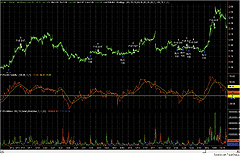--Mark Mills
EasyLanguage Questions Forum
A subsidiary of TradeStation Group, Inc.TRADESTATION: CHART SENTIMENT INDEX

Viktor Likhovidov's article in this issue, "Index Of Chart Sentiment," describes a method of determining sentiment from a price chart via candlestick interpretation.

The EasyLanguage implementation I'll give here is based on the CandleCode function developed by Ramesh Dhingra at TradeStation Securities, which he presented in the March 2001 Traders' Tips column. That function is not shown here, but will be part of the .eld file available for download from TradeStationWorld.

Likhovidov's sentiment characterizations have been converted into four zones, each with their own color. The EasyLanguage implementation given here follows the rules prescribed in Likhovidov's article, with one exception: Instead of manually drawing linear zone boundaries to define extreme sentiment zones, the code draws Bollinger Bands above and below the sentiment average (Figure 2). This allows the user to automate the categorization of sentiment. Likhovidov leaves it to the user to define additional confirmation to signals in the up-pulse and down-pulse zones. For this example, the confirmation was an overbought/oversold stochastic reading.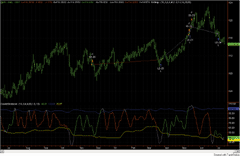FIGURE 2: TRADESTATION, INDEX OF CHART SENTIMENT. Here's a sample TradeStation chart displaying the chart sentiment index described by Viktor Likhovidov in this issue.
Indicator: CandleSentiment
inputs:
``` BBLength( 10 ),
BBNumDevs( .5 ),
AvgLength1( 4 ),
AvgLength2( 4 ),
BBILength( 60 ),
NumDevsUp( 1.5 ),
NumDevsDn( 1.5 ) ;
variables:
Index( 0 ),
Avg( 0 ),
SDev( 0 ),
UpperBand( 0 ),
LowerBand( 0 ),
Zone( 0 ) ;
Index = Average( Average(
CandleCode( BBLength, BBNumDevs ), AvgLength1 ),
AvgLength2 ) ;
if CurrentBar > ( BBILength + BBLength ) * 2 then
begin
Avg = Average( Index, BBILength ) ;
SDev = StandardDev( Index, BBILength, 1 ) ;
UpperBand = Avg + NumDevsUp * SDev ;
LowerBand = Avg - NumDevsDn * SDev ;
Plot1( Index, "CCode" ) ;
Plot2( UpperBand, "UB" ) ;
Plot3( LowerBand, "LB" ) ;
if Index <= UpperBand
and Index > UpperBand
then
Zone = 3  { UpPulse }
else if Index >= UpperBand
and Index < UpperBand
then
Zone = 6  { Bear }
else if Index >= LowerBand
and Index < LowerBand
then
Zone = 5  { DownPulse }
else if Index <= LowerBand
and Index > LowerBand
then
Zone = 4 ; { Bull }
SetPlotColor( 1, Zone ) ;
end ;```
Strategy: CandleSentimentStrat
inputs:
``` BBLength( 10 ),
BBNumDevs( .5 ),
AvgLength1( 4 ),
AvgLength2( 4 ),
BBILength( 60 ),
NumDevsUp( 1.5 ),
NumDevsDn( 1.5 ) ;
inputs:
Length( 14 ),
OverSold( 20 ),
OverBought( 80 ) ;
variables:
oFastK( 0 ),
oFastD( 0 ),
oSlowK( 0 ),
oSlowD( 0 ) ;
variables:
Index( 0 ),
Avg( 0 ),
SDev( 0 ),
UpperBand( 0 ),
LowerBand( 0 ),
Zone( 0 ) ;
Index = Average( Average( CandleCode(
BBLength, BBNumDevs ), AvgLength1 ), AvgLength2 ) ;
if CurrentBar > ( BBILength + BBLength ) * 2 then
begin
Avg = Average( Index, BBILength ) ;
SDev = StandardDev( Index, BBILength, 1 ) ;
UpperBand = Avg + NumDevsUp * SDev ;
LowerBand = Avg - NumDevsDn * SDev ;
if Index <= UpperBand
and Index > UpperBand
then
Zone = 3  { UpPulse }
else if Index >= UpperBand
and Index < UpperBand
then
Zone = 6  { Bear }
else if Index >= LowerBand
and Index < LowerBand
then
Zone = 5  { DownPulse }
else if Index <= LowerBand
and Index > LowerBand
then
Zone = 4 ; { Bull }
Value1 = Stochastic( H, L, C, Length, 3, 3, 1,
oFastK, oFastD, oSlowK, oSlowD ) ;
if CurrentBar > 2
and oSlowK crosses over oSlowD
and Zone = 4
then
Buy ( "LE-Z4" ) next bar at market ;
if CurrentBar > 2
and oSlowK crosses under oSlowD
and Zone = 6
then
Sell Short ( "SE-Z6" ) next bar at market ;
if CurrentBar > 2
and oSlowK crosses over oSlowD
and Zone = 3
then
Buy ( "LE-Z3" ) next bar at market ;
if CurrentBar > 2
and oSlowK crosses under oSlowD
and Zone = 3
and oSlowK > OverBought
then
Sell Short ( "SE-Z3" ) next bar at market ;
if CurrentBar > 2
and oSlowK crosses over oSlowD
and Zone = 5 and oSlowK < OverSold
then
Buy ( "LE-Z5" ) next bar at market ;
if CurrentBar > 2
and oSlowK crosses under oSlowD
and Zone = 5
then
Sell Short ( "SE-Z5" ) next bar at market ;
end ;```

This indicator and strategy code will be available for download from the EasyLanguage Exchange at www.tradestationworld.com. The filename is "CandleSentiment.eld."

--Mark Mills
EasyLanguage Questions Forum
A subsidiary of TradeStation Group, Inc.METASTOCK: DETECTING BREAKOUTS INTRADAY

Editor's note: The MetaStock code for Markos Katsanos' article "Detecting Breakouts In Intraday Charts" is given in the article.METASTOCK: CHART SENTIMENT INDEX

Viktor Likhovidov's article "Index Of Chart Sentiment" builds on his previous articles presenting CandleCode (November 1999, April 2001). In this month's article, Likhovidov gives the MetaStock formula for the double-smoothed symmetric variant of CandleCode (IndSent - CW), which requires the formulas he introduced in the previous articles.

These formulas are given here. These formulas are all used by the CandleWeight formula through an Fml() function. It is very important that the names are typed exactly the same everywhere you see them. If either spacing or capitalization is off, the referencing formula will give errors.

First, create the following three formulas in MetaStock. To do so, go to Tools | Indicator Builder. Click New and enter the name for the formula. Then click in the larger window and enter the actual formula. Click OK. If the formula was entered with no syntax errors, you will be returned to the Indicator Builder, ready to create the next formula.

```Name: body
Formula:
Abs(OPEN-CLOSE)

Name: lshd
Formula:
If(CLOSE>=OPEN, OPEN-LOW, CLOSE-LOW)

Name: ushd
Formula:
If(CLOSE>=OPEN, HIGH-CLOSE, HIGH-OPEN)```

After you have created the first three, you can now create the next six formulas. Since they reference the first three, those had to be made first.

```Name: ThBot_b
Formula:
BBandBot( Fml( "body") ,55,E,0.5)

Name: ThBot_l
Formula:
BBandBot( Fml( "lshd") ,55,E,0.5)

Name: ThBot_u
Formula:
BBandBot( Fml( "ushd") ,55,E,0.5)

Name: ThTop_b
Formula:
BBandTop( Fml( "body") ,55,E,0.5)

Name: ThTop_l
Formula:
BBandTop( Fml( "lshd") ,55,E,0.5)

Name: ThTop_u
Formula:
BBandTop( Fml( "ushd") ,55,E,0.5)```
The CandleWeight formula can now be created. After it is entered, you can create the IndSent - CW formula.

```Name: CandleWeight
Formula:
If(C=O,1,0)*If(Fml("ushd") >= Fml("lshd"),64,-64)+
If(C=O,0,1)*If(C>O,1,-1)*(If(Fml("body")<= Fml("ThBot_b"),80,0)+
If( Fml("body")> Fml("ThBot_b") AND Fml("body")<=Fml("ThBot_b"),96,0)+
If(Fml("body")> Fml("ThTop_b"),112,0))+
If(C>=O,-4,4)*(If(Fml("lshd")=0,3,0)+
If(Fml("lshd")< Fml("ThBot_l") AND  Fml("lshd")>0,2,0)+
If( Fml("lshd")> Fml("ThBot_l") AND Fml("lshd")<= Fml("ThTop_l") AND Fml("lshd")>0,1,0))+
If(C>=O,1,-1)*(If( Fml("ushd")>0 AND Fml("ushd")<= Fml("ThBot_u"),4,0)+
If(Fml("ushd")> Fml("ThBot_u") AND Fml("ushd")<=Fml("ThTop_u"),8,0)+
If(Fml("ushd")> Fml("ThTop_u"),12,0))```

After plotting the IndSent - CW indicator, you can quickly plot the four lines with the standard error channel line study (Figure 3). Plot it once, right-click on it, and change the properties to the desired number of errors. Then you can plot it again for the second number of errors. Figure 3 uses 1.5 and 1 for the number of errors.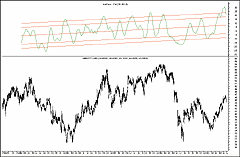FIGURE 3: METASTOCK, INDEX OF CHART SENTIMENT. After plotting the IndSent - CW indicator, you can quickly plot the four lines with the standard error channel line study. Change the properties to the desired number of errors. Then you can plot it again for the second number of errors. This chart uses 1.5 and 1 for the number of errors.
-- William Golson, Equis Support
Equis International, www.equis.com
GO BACKAMIBROKER: DETECTING BREAKOUTS INTRADAY

In "Detecting Breakouts In Intraday Charts," Markos Katsanos presents a modification to his finite volume elements (FVE) indicator, which he originally presented in the April 2003 S&C. In this month's article, Katsanos also shows how to construct a trading system based on this indicator. The volatility-modified FVE indicator, the color-coded volume bar chart, and the FVI trading system can be easily reproduced in AmiBroker using its native AFL language.

Listing 1 shows ready-to-use code that can be applied in both Indicator Builder and Automatic Analysis windows. To backtest the system, simply enter the formula given here into the formula field of the Automatic Analysis window and press the "Backtest" button. AmiBroker allows you to backtest both a fixed-position-size trading (\$10,000 per trade) scheme and a profit/loss compounding scheme.

LISTING 1
/* Volatility modified FVE chart
```** plus Volatility color-coded Volume chart + trading system
** TJ for S&C Traders Tips Sep 2003,
*/
Period = Param("Period for FVE", 24, 5, 80, 1 );
Coeff = Param("Coeff for Cutoff", 0.1, 0, 2, 0.01 );
intra=log(H)-log(L);
Vintra = StDev(intra, period );
inter = log(Avg)-log(Ref(Avg,-1));
Vinter = StDev(inter,period);
Cutoff = Coeff * (Vinter+Vintra)*C;
MF = C- (H+L)/2 + Avg - Ref( Avg, -1 );
VC = IIf( MF > Cutoff, V,
IIf( MF < -Cutoff, -V, 0 ));
FVE = 100 * Sum( VC, Period )/(MA( V, Period ) * Period );
Plot( FVE, "Modified FVE", colorYellow, styleThick );
// volatility color-coded volume bars:
BarColor =
IIf( MF > Cutoff,  colorGreen, /* up volume */
IIf( MF < -Cutoff, colorRed, /* down volume */
colorBlue /*otherwise*/ ));
Plot( Volume, "Volume", BarColor, styleHistogram | styleThick | styleOwnScale );
PositionSize = 10000; // fixed position size - remove to enable compounding
RoundLotSize = 10;
BuyPrice = Close; SellPrice = Close;
LinRegAngleFVE = RAD_TO_DEG * atan( LinRegSlope( FVE, 20 ) );
Buy = FVE < 10 AND
FVE > -20 AND
LinRegAngleFVE > 30 AND
FVE > EMA( FVE, 40 ) AND
LinRegSlope( C, 30 ) < Ref(C, -30 ) *0.6/100 AND
LinRegSlope( C, 30 ) > -Ref( C, -30 ) * 0.3/100;
Sell = LinRegAngleFVE < -30;```

A downloadable version of this formula is available from AmiBroker's website. Figure 4 shows the FVE and FVI system plotted on Atmel Corp.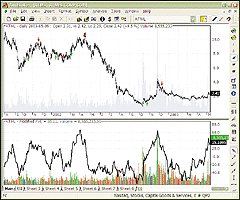FIGURE 4: AMIBROKER, FVE AND FVI. A daily Atmel Corporation chart is shown with buy and sell arrows generated by the FVI trading system (upper pane) and the modified FVI plotted over a color-coded volume bar chart (lower pane).
--Tomasz Janeczko, AmiBroker.com
www.amibroker.comAMIBROKER: INDEX OF CHART SENTIMENT

In "Index Of Chart Sentiment," author Viktor Likhovidov presents yet another modification of his CandleCode indicator, introduced in STOCKS & COMMODITIES in November 1999. The index of chart sentiment (ICS) is a double-smoothed CandleWeight indicator that was introduced by the same author back in 2001. Implementation of ICS is relatively simple in AmiBroker Formula Language (Figure 5). To recreate the indicator in AmiBroker, select "Indicator Builder" from the Analysis menu and enter the following code: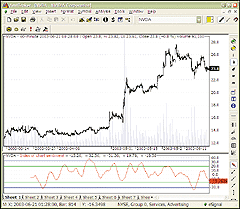FIGURE 5: AMIBROKER, INDEX OF CHART SENTIMENT. This AmiBroker chart shows the index of chart sentiment (lower pane) applied to an hourly chart of NVDA. Oversold/overbought boundary lines are automatically generated using the standard error function.
```LISTING 1
Body = abs( Open - Close );
Lshd = IIf( Close >= Open, Open - Low, Close-Low );
Ushd = IIf( Close >= Open, High - Close, High - Open );
ThBotB =BBandBot( Body,55,0.5);
ThTopB =BBandTop( Body,55,0.5);
ThBotL =BBandBot( Lshd,55,0.5);
ThTopL =BBandTop( Lshd,55,0.5);
ThBotU =BBandBot( Ushd,55,0.5);
ThTopU =BBandTop( Ushd,55,0.5);
CandleWeight =
( Close == Open ) * IIf( Ushd >= Lshd, 64, -64)
+
( Close != Open ) * IIf( Close > Open, 1, -1 ) *
(
IIf( body <= ThBotB, 80, 0 ) +
IIf( body > ThBotB AND body <= ThTopB, 96, 0 ) +
IIf( body > ThTopB, 112, 0 )
)
+
IIf( Close >= Open, -4, 4 ) *
(
IIf( lshd = 0, 3, 0 ) +
IIf( lshd < ThBotL AND lshd > 0, 2,0 ) +
IIf( lshd > ThBotL AND lshd <= ThTopL AND lshd > 0, 1, 0 )
)
+
IIf( Close >= Open, 1, -1 ) *
(
IIf( ushd > 0 AND ushd <= ThBotU, 4, 0 ) +
IIf( ushd > ThBotU AND ushd <= ThTopU , 8, 0 ) +
IIf( ushd > ThTopU, 12, 0 )
);
ICS = MA( MA( CandleWeight, 24 ), 24 );
Plot( ICS, "Index of chart sentiment", colorRed );
ChannelWidth = LastValue( StdErr( ICS, BarCount/2 ));
Center= LastValue(Highest(ICS)+Lowest(ICS))/2;
Plot( Center + 2.5 *ChannelWidth, "", colorBlue, styleNoLabel );
Plot( Center - 2.5 *ChannelWidth, "", colorBlue , styleNoLabel);
Plot( Center + 1.5*ChannelWidth, "", colorGreen, styleNoLabel );
Plot( Center - 1.5 * ChannelWidth, "", colorGreen, styleNoLabel );
GraphXSpace=2;```
--Tomasz Janeczko, AmiBroker.com
www.amibroker.com
GO BACKeSIGNAL: DETECTING BREAKOUTS INTRADAY

These eSignal formulas are based on "Detecting Breakouts In Intraday Charts" by Markos Katsanos in this issue.

```/*******************************************************************
Description : This Indicator plots the Finite Volume Elements
Provided By : TS Support, LLC for eSignal
********************************************************************/
function preMain(){
setStudyTitle("Finite Volume Elements");
setCursorLabelName("FVI",0);
setDefaultBarFgColor(Color.green,0);
setComputeOnClose();
setDefaultBarThickness(2);
}
var FVE = 0;
function main(Period){
if(Period == null)
Period = 22;
var MF = 0, vlm = 0, Sum = 0;
MF = close() - (high() + low()) / 2
+ (high() + low() + close()) / 3
- (high(-1) + low(-1) + close(-1)) / 3;
if(MF > .3 * close() / 100)
vlm = volume();
else if(MF < -.3 * close() / 100)
vlm = - volume();
else
vlm = 0;
for(i = - Period + 1; i <= 0; i++)
Sum += volume(i);
VolumeMA = Sum / Period;
if(getBarState() == BARSTATE_NEWBAR)
FVE += ((vlm / VolumeMA) / Period) * 100;
return FVE;
}
/*******************************************************************
Description : FVE Strategy
Provided By : TS Support, LLC for eSignal
********************************************************************/
/* System Testing Options:
* Fixed Dollars per trade: \$10,000
* Commissions: Entry \$10, Exit: \$10
* Positions: Longs only
* Number of shares rounded to the nearest 10 shares
*/
function preMain(){
setStudyTitle("FVE Strategy");
setComputeOnClose();
setPriceStudy(true);
}
var EMA_1 = 0;
VolumePlusMinusArray = new Array();
FVEArray = new Array();
function main(Samples,FVEenterl,FVEenteru,MA,LRPeriod,Bangle,Sangle,LRC,UB,LB,BarToExitOn){
if(Samples == null) Samples = 50;
if(FVEenterl == null) FVEenterl = -20;
if(FVEenteru == null) FVEenteru = 10;
if(MA == null) MA = 40;
if(LRPeriod == null) LRPeriod = 20;
if(LRPeriod == null) LRPeriod = 20;
if(Bangle == null) Bangle = 30;
if(Sangle == null) Sangle = -30;
if(LRC == null) LRC = 30;
if(UB == null) UB = .1;
if(LB == null) LB = -.5;
if(BarToExitOn == null) BarToExitOn = 70;
var TP = 0, TP1 = 0, MF = 0, Cintra = .1, Cinter = .1, CutOff = 0, VolumePlusMinus = 0,
FVE = 0, Fvesum = 0, FveFactor = 0, Intra = 0, Inter = 0, Vintra = 0, Vinter = 0,
i = 0, VolSum = 0; K = 2 / (MA + 1), EMA = 0, vHL = high() - low(), vVar = vHL * 0.25,
vAddVar = vVar * 0.35, Condition1 = false, Condition2 = false,
Condition3 = false, Condition4 = false, Condition5 = false;
IntraArray = new Array();
InterArray = new Array();
for(i = 0; i < Samples; i++){
IntraArray[i] = Math.log(high(-i)) - Math.log(low(-i));
InterArray[i] = Math.log((high(-i) + low(-i) + close(-i))/3)
- Math.log((high(-i-1)+ low(-i-1) + close(-i-1))/3);
}
TP = (high() + low() + close())/3;
TP1 = (high(-1)+ low(-1) + close(-1))/3;
Intra = Math.log(high()) - Math.log(low());
Vintra = StandardDev(IntraArray,Samples);
Inter = Math.log(TP) - Math.log(TP1);
Vinter = StandardDev(InterArray,Samples);
CutOff = Cintra * Vintra + Cinter * Vinter;
MF = (close() - (high() + low())/2)+ TP - TP1;
if(MF > CutOff * close())
FveFactor = 1;
else if(MF < -1 * CutOff * close())
FveFactor = -1;
else
FveFactor=0;
VolumePlusMinus = volume() * FveFactor;
for(i = Samples - 1; i > 0; i--)
VolumePlusMinusArray[i] = VolumePlusMinusArray[i - 1];
VolumePlusMinusArray = VolumePlusMinus;
for(i = 0; i < Samples; i++){
Fvesum += VolumePlusMinusArray[i];
VolSum += volume(-i);
}
if(VolumePlusMinusArray[Samples - 1] != null){
FVE = (Fvesum / VolSum) * 100;
for(i = LRPeriod - 1; i > 0; i--)
FVEArray[i] = FVEArray[i - 1];
FVEArray = FVE;
EMA = K * FVE  + (1 - K) * EMA_1;
if (getBarState() == BARSTATE_NEWBAR)
EMA_1 = EMA;
}
else
return null;
if(FVEArray[LRPeriod - 1] != null){
if(FVE > FVEenterl && FVE < FVEenteru)
Condition1 = true;
if(LinearReg(FVEArray,LRPeriod,"AngleFC",LRC-1) > Bangle)
Condition2 = true;
if(FVE > EMA)
Condition3 = true;
if(LinearReg(close(0,-LRC),LRC,"Slope",LRC-1) < UB * LinearReg(close
(0,-LRC),LRC,"Value",LRC-1) / 100 &&  LinearReg(close(0,-LRC),LRC,
"Slope",LRC-1) > LB * LinearReg(close(0,-LRC),LRC,"Value",LRC-1) / 100)
Condition4 = true;
if(LinearReg(FVEArray,LRPeriod,"AngleFC",LRC-1) < Sangle)
Condition5 = true;
if(!Strategy.isInTrade() && Condition1 && Condition2 && Condition3 && Condition4){
drawShapeRelative(0, low() - vVar, Shape.UPARROW, "", Color.lime,
Color.lime, Text.BOLD | Text.ONTOP, null, null, "buyTxt" + getValue("time"));
}
if(Condition5){
Strategy.doShort("FVE EXIT", Strategy.CLOSE, Strategy.THISBAR);
drawShapeRelative(0, high() + vVar, Shape.DOWNARROW, "", Color.red,
null, "sellShp" + getValue("time"));
drawTextRelative(-1, high() + (vVar + vAddVar), "Short", Color.black,
Color.red, Text.BOTTOM | Text.BOLD | Text.ONTOP, null, null, "buyTxt"
+ getValue("time"));
}
Strategy.doShort("TimeBarsLX", Strategy.CLOSE, Strategy.THISBAR);
drawShapeRelative(0, high() + vVar, Shape.DOWNARROW, "", Color.red,
null, "sellShp" + getValue("time"));
drawTextRelative(-1, high() + (vVar + vAddVar), "Short", Color.black,
Color.red, Text.BOTTOM | Text.BOLD | Text.ONTOP, null, null, "buyTxt"
+ getValue("time"));
}
return LinearReg(close(0,-LRC),LRC,"Slope",LRC-1);
}
else
return null;
}
function StandardDev(Array,Length,Type){
var i;
var vSum = 0;
var SumSqr = 0;
var StdDev = 0;
for(i = 0; i < Length; i++)
vSum += Array[i];
if(Length != 0)
for(i = 0; i < Length; i++)
SumSqr += (Array[i] - vSum / Length) * (Array[i] - vSum / Length);
StdDev = Math.sqrt(SumSqr / Length);
return StdDev;
}
function LinearReg(Array,Length,Type,TargetB){
var i = 0, num1 = 0, num2 = 0, SumBars = 0, SumSqrBars = 0, SumY = 0,
Sum1 = 0, Sum2 = 0, Slope = 0, Intercept = 0;
if(Length == 0)
return 0;
SumBars = Length * (Length - 1) * .5;
SumSqrBars = (Length - 1) * Length * (2 * Length - 1) / 6;
Sum1 = 0;
for(i = 0; i < Length; i++){
Sum1 += i * Array[i];
SumY += Array[i];
}
Sum2 = SumBars * SumY;
Num1 = Length * Sum1 - Sum2;
Num2 = SumBars * SumBars - Length * SumSqrBars;
if(Num2 != 0)
Slope = Num1 / Num2;
else
Slope = 0;
if(Type == "AngleFC")
return Math.atan(Slope);
else if(Type == "Slope")
return Slope;
else if(Type == "Value"){
Intercept = (SumY - Slope * SumBars) / Length;
return Intercept + Slope * (Length - 1 - TargetB);
}
}
/*********************************************************************
Description : This Indicator plots the volatility-modified FVE formula
Provided By : TS Support, LLC for eSignal
********************************************************************/
function preMain(){
setStudyTitle("Volatility Finite Volume Elements");
setCursorLabelName("FVI",0);
setDefaultBarFgColor(Color.green,0);
setCursorLabelName("FVI EMA",1);
setDefaultBarFgColor(Color.blue,1);
setDefaultBarThickness(2);
setComputeOnClose();
}
var EMA_1 = 0;
VolumePlusMinusArray = new Array();
function main(Samples,Perma,Cintra,Cinter){
if(Samples == null)
Samples = 22;
if(Perma == null)
Perma = 40;
if(Cintra == null)
Cintra = .1;
if(Cinter == null)
Cinter = .1;
var TP = 0, TP1 = 0, VolumePlusMinus = 0, FVE = 0, Fvesum = 0, VolSum = 0,
FveFactor = 0, Intra = 0, Inter = 0, Vintra = 0, Vinter = 0, CutOff = 0,
i = 0, K = 2 / (Perma + 1), EMA = 0;
IntraArray = new Array();
InterArray = new Array();
for(i = 0; i < Samples; i++){
IntraArray[i] = Math.log(high(-i)) - Math.log(low(-i));
InterArray[i] = Math.log((high(-i) + low(-i) + close(-i))/3)
- Math.log((high(-i-1)+ low(-i-1) + close(-i-1))/3);
}
TP = (high() + low() + close())/3;
TP1 = (high(-1)+ low(-1) + close(-1))/3;
Intra = Math.log(high()) - Math.log(low());
Vintra = StandardDev(IntraArray,Samples);
Inter = Math.log(TP) - Math.log(TP1);
Vinter = StandardDev(InterArray,Samples);
CutOff = Cintra * Vintra + Cinter * Vinter;
MF = (close() - (high() + low())/2)+ TP - TP1;
if(MF > CutOff * close())
FveFactor = 1;
else if(MF < -1 * CutOff * close())
FveFactor = -1;
else
FveFactor=0;
VolumePlusMinus = volume() * FveFactor;
for(i = Samples - 1; i > 0; i--)
VolumePlusMinusArray[i] = VolumePlusMinusArray[i - 1];
VolumePlusMinusArray = VolumePlusMinus;
for(i = 0; i < Samples; i++){
Fvesum += VolumePlusMinusArray[i];
VolSum += volume(-i);
}
if(VolumePlusMinusArray[Samples - 1] != null){
FVE = (Fvesum / VolSum) * 100;
EMA = K * FVE  + (1 - K) * EMA_1;
if (getBarState() == BARSTATE_NEWBAR)
EMA_1 = EMA;
return new Array(FVE,EMA);
}
return null;
}
function StandardDev(Array,Length){
var i;
var vSum = 0;
var SumSqr = 0;
var StdDev = 0;
for(i = 0; i < Length; i++)
vSum += Array[i];
if(Length != 0)
for(i = 0; i < Length; i++)
SumSqr += (Array[i] - vSum / Length) * (Array[i] - vSum / Length);
StdDev = Math.sqrt(SumSqr / Length);
return StdDev;
}
/*************************************************************************
Description : This Indicator plots the volatility color-coded volume bar FVE formula
Provided By : TS Support, LLC for eSignal
**************************************************************************/
function preMain(){
setStudyTitle("Volatility color-coded Volume Bar Formula");
setCursorLabelName("VBF",0);
setDefaultBarFgColor(Color.green,0);
setCursorLabelName("VBF EMA",1);
setDefaultBarFgColor(Color.blue,1);
setDefaultBarThickness(2);
setComputeOnClose();
}
var VMA = null;
var EMA_1 = 0;
VolumePlusMinusArray = new Array();
if(AvgLength == null)
AvgLength = 50;
if(Cintra == null)
Cintra = .1;
if(Cinter == null)
Cinter = .1;
if(Samples== null)
Samples= 22;
if(VMA == null)
VMA = new MAStudy(AvgLength, 0, "Volume", MAStudy.SIMPLE);
var TP = 0, TP1 = 0,  MF = 0, Intra = 0, Inter = 0, Vintra = 0,
Vinter = 0, CutOff = 0, i = 0;
IntraArray = new Array();
InterArray = new Array();
for(i = 0; i < Samples; i++){
IntraArray[i] = Math.log(high(-i)) - Math.log(low(-i));
InterArray[i] = Math.log((high(-i) + low(-i) + close(-i))/3)
- Math.log((high(-i-1)+ low(-i-1) + close(-i-1))/3);
}
TP = (high() + low() + close())/3;
TP1 = (high(-1)+ low(-1) + close(-1))/3;
Intra = Math.log(high()) - Math.log(low());
Vintra = StandardDev(IntraArray,Samples);
Inter = Math.log(TP) - Math.log(TP1);
Vinter = StandardDev(InterArray,Samples);
CutOff = Cintra * Vintra + Cinter * Vinter;
MF = (close() - (high() + low())/2)+ TP - TP1;
if(MF > CutOff * close())
setBarFgColor(Color.green);
else if(MF < -1 * CutOff * close())
setBarFgColor(Color.red);
else
setBarFgColor(Color.blue);
return new Array(volume(),VMA.getValue(MAStudy.MA));
}
function StandardDev(Array,Length){
var i;
var vSum = 0;
var SumSqr = 0;
var StdDev = 0;
for(i = 0; i < Length; i++)
vSum += Array[i];
if(Length != 0)
for(i = 0; i < Length; i++)
SumSqr += (Array[i] - vSum / Length) * (Array[i] - vSum / Length);
StdDev = Math.sqrt(SumSqr / Length);
return StdDev;
}```
--eSignal, a division of Interactive Data Corp.
800 815-8256, www.esignal.com
GO BACKWEALTH-LAB: DETECTING BREAKOUTS INTRADAY

The volatility-modified FVE is available as a custom indicator at the Wealth-Lab.com website and in our Wealth-Lab Developer desktop software. The script below implements the daily bar trading system based on VolModFve as described in Markos Katsanos' article in this issue, "Detecting Breakouts In Intraday Charts."

You can test this system on any US stock at the Wealth-Lab.com website, or use Wealth-Lab Developer to evaluate the system on a complete portfolio of stocks and/or futures.

The VolModFve custom indicator does a bit of additional work behind the scenes. It records whether each bar contributed positive volume, negative volume, or zero to the indicator. The information is stored in a specially named data series, which is available to any scripts that use the indicator. In our sample script, we access this information by using the FindNamedSeries function to return the data series named "ColorCode." Then, based on the contents of this data series, we color the volume bars red, green, or silver (Figure 7).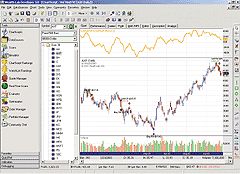FIGURE 7: WEALTH-LAB VOLUME-MODIFIED FVE. This sample Wealth-Lab chart plots the VolModFve. The volume bars are colored red, green, or silver based on the contents of the data series named "ColorCode."
```{\$I 'VolModFVE'}
var Bar, ColorCode: integer;
var VolModFVEPane: integer;
var VolModFVE1: integer;

{ Plot the volatility-modified FVE }
VolModFVEPane := CreatePane( 75, true, true );
VolModFVE1 := VolModFVESeries( 22,0.1 );
PlotSeriesLabel( VolModFVE1, VolModFVEPane, 950, #Thick, 'VolModFVE1=VolModFVE(22,0.1)' );

{ Color-code the volume bars }
ColorCode := FindNamedSeries( 'ColorCode' );
for Bar := 22 to BarCount - 1 do
begin
if @ColorCode[Bar] = 1 then
SetSeriesBarColor( Bar, #Volume, #Green )
else if @ColorCode[Bar] = -1 then
SetSeriesBarColor( Bar, #Volume, #Red )
else
SetSeriesBarColor( Bar, #Volume, #Silver );
end;

{ Implement the daily bar trading system }
for Bar := 22 to BarCount - 1 do
begin
if MarketPosition = 0 then
begin
if VolModFVE( Bar, 22, 0.1 ) < 10 then
if VolModFVE( Bar, 22, 0.1 ) > -20 then
if LinearRegSlope( Bar, VolModFVE1, 20 ) > 0.58 then
if VolModFVE( Bar, 22, 0.1 ) > EMA( Bar, VolModFVE1, 40 ) then
if LinearRegSlope( Bar, #Close, 30 ) < PriceClose( Bar - 30 ) * 0.6 / 100 then
BuyAtMarket( Bar + 1, '' );
end
else
begin
if LinearRegSlope( Bar, VolModFVE1, 20 ) < -0.58 then
SellAtMarket( Bar + 1, LastPosition, '' );
end;
end;```

--Dion Kurczek, Wealth-Lab, Inc.
www.wealth-lab.com
GO BACKWEALTH-LAB: INDEX OF CHART SENTIMENT

After Viktor Likhovidov introduced his CandleCode indicator back in the November 1999 issue of S&C, we made it available at the Wealth-Lab.com system-testing website, as well as in our Wealth-Lab Developer desktop software.

The index of chart sentiment (ICS) described in this month's article by Viktor Likhovidov is based on a double-smoothed CandleCode indicator, and is very easy to construct using our WealthScript language. The script shown here calculates and plots the ICS, as well as a Bollinger Band channel around the indicator. The background of the chart is colored green for bullish zones and red for bearish zones. An eight-period RSI is also plotted as described in the article (Figure 8).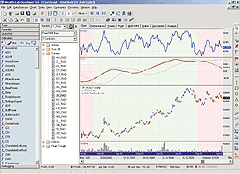FIGURE 8: WEALTH-LAB, INDEX OF CHART SENTIMENT. This sample Wealth-Lab chart plots Viktor Likhovidov's index of chart sentiment (ICS) based on a double-smoothed CandleCode indicator. An eight-period RSI is also plotted in the chart, as well as a Bollinger Band channel around the indicator. The background of the chart is colored green for bullish zones and red for bearish zones.
```{\$I 'CandleCode'}
var ICS, CC, RSI8, ICSPane, RSIPane: integer;
var BBU, BBL: integer;

{ Calculate Indicators }
RSI8 := RSISeries( #Close, 8 );
CC := CandleCodeSeries;
ICS := SMASeries( SMASeries( CC, 24 ), 24 );
BBL := BBandLowerSeries( ICS, 20, 2 );
BBU := BBandUpperSeries( ICS, 20, 2 );

{ Plot them }
ICSPane := CreatePane( 100, true, true );
PlotSeriesLabel( ICS, ICSPane, #Maroon, #Thick, 'ICS' );
PlotSeriesLabel( BBU, ICSPane, #Silver, #Thick, 'Upper BBand' );
PlotSeriesLabel( BBL, ICSPane, #Silver, #Thick, 'Lower BBand' );
RSIPane := CreatePane( 100, true, true );
PlotSeriesLabel( RSI8, RSIPane, #Blue, #Thick, 'RSI(8)' );

{ Determine Bullish and Bearish zones }
var Bar: integer;
var Bullish, Bearish: boolean;
for Bar := 50 to BarCount - 1 do
begin
if Bullish then
begin
if @ICS[Bar] < @BBL[Bar] then
Bullish := false;
end
else
begin
if @ICS[Bar] > @BBU[Bar] then
Bullish := true;
end;
if Bullish then
SetBackgroundColor( Bar, #GreenBkg )
else
SetBackgroundColor( Bar, #RedBkg );
end;```
--Dion Kurczek, Wealth-Lab, Inc.
www.wealth-lab.comNEUROSHELL TRADER: DETECTING BREAKOUTS INTRADAY

To implement Markos Katsanos' breakout-detection trading system using the modified finite volume elements (FVE) indicator in NeuroShell Trader, you should first create the modified FVE indicator in a chart and then create a NeuroShell Trading Strategy based on that indicator.

To create the modified finite volume elements indicator, select "New Indicator ..." from the Insert menu and use the Indicator Wizard to create each of the following:

```TYPICAL:
Avg3 (High, Low, Close)
MIDPOINT:
Avg2 (High, Low)
MF:
Add2 ( Sub (Close, MIDPOINT), Momentum (TYPICAL, 1) )
INTRA:
Sub ( Ln ( High ), Ln ( Low ) )
INTER:
Momentum ( Ln ( TYPICAL ), 1 )
CUTOFF:
Add2 ( Mult2 ( 0.1, StndDev (INTRA, 50) ), Mult2 ( 0.1, StndDev(INTER,50) ) )
INEQUALITY:
IfThenElseIfThen ( A>B ( MF, Mult2 (CUTOFF, Close) ),
Volume, A<B ( MF, Mult3 (-1, CUTOFF, Close) ),
Mult (Volume, -1), 0 )
MFVE:
Mult ( Divide ( Divide ( Sum (INEQUALITY, 22), MovAvg (Volume, 22) ), 22 ) , 100 )
To create the modified finite volume elements trading system,
the following long entry and exit conditions in the appropriate
locations of the Trading Strategy Wizard:
Generate a buy long MARKET order if ALL of the following are true:
A<B<C ( -20, MFVE, 10 )
A>B ( InvTan ( LinRegSlope (MFVE, 20)) , 30 )
A>B ( MFVE, ExpAvg (MFVE, 40 ) )
A<B<C ( -30, %Change(LinRegSlope (Close,30), 30 ), 60 )
Generate a sell long MARKET order if ONE of the following is true:
A<B ( InvTan ( LinRegSlope (MFVE, 20)) , -20 )

If you have NeuroShell Trader Professional, you can also choose whether the system parameters should be optimized. After backtesting the trading strategy, use the "Detailed Analysis ..." button to view the backtest and trade-by-trade statistics for the modified finite volume elements system.

Users of NeuroShell Trader can go to the STOCKS & COMMODITIES section of the NeuroShell Trader free technical support website to download a sample chart that includes the modified finite volume elements custom indicator and modified Fve trading system (Figure 9).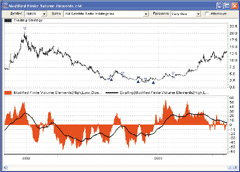FIGURE 9: NEUROSHELL TRADER, FVE. Here's a sample NeuroShell Trader chart demonstrating the modified finite volume elements indicator.

--Marge Sherald, Ward Systems Group, Inc.
301 662-7950, sales@wardsystems.com
www.neuroshell.com
GO BACKNEUROSHELL TRADER: INDEX OF CHART SENTIMENT

The indicator that Viktor Likhovidov describes in this issue is easily implemented in NeuroShell Trader using a simple moving average and the CandleWeight indicator that Likhovidov described in his March 2001 article in S&C.  The CandleWeight indicator is quite complex and was coded in a standard programming language rather than NeuroShell's Indicator Wizard. NeuroShell Trader can directly call external code written in C, C++, Power Basic, and Delphi.

In addition to coding the indicator CandleWeight, we have also coded the indicator CandleCode. The CandleCode indicator is described in Viktor Likhovidov's original "Coding Candlesticks" article in the November 1999 issue of S&C.  A sample chart of the CandleWeight and CandleCode indicators is in Figure 10.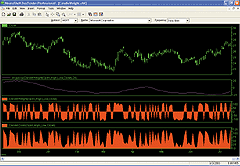FIGURE 10: NEUROSHELL TRADER, INDEX OF CHART SENTIMENT. Here is a daily chart of the CandleWeight indicator in NeuroShell Trader.

Users of NeuroShell Trader can go to the STOCKS & COMMODITIES section of the NeuroShell Trader free technical support website to download the CandleWeight and CandleCode indicators. After downloading the custom indicators, you can insert them into charts, predictions, and trading strategies using the Indicator Wizard.

--Marge Sherald, Ward Systems Group, Inc.
301 662-7950, sales@wardsystems.com
www.neuroshell.com
GO BACKNEOTICKER: DETECTING BREAKOUTS INTRADAY

The two indicators and one trading strategy presented in "Detecting Breakouts In Intraday Charts" by Markos Katsanos can be implemented in NeoTicker using formula indicators.

Starting with the volatility-modified Fve formula, create a formula indicator and name it fvevol with four parameters: Samples, Perma, Cintra, and Cinter (Listing 1). Fvevol has four plots in total: The first plot is the FVE values, the second plot is the EMA of FVE, the third plot is the zero line, and the fourth plot is the alerts, shown as dots along the zero line (Figure 11). Note this indicator uses a tangent of 30 degrees to compare the linear regression slope instead of comparing the linear regression angle.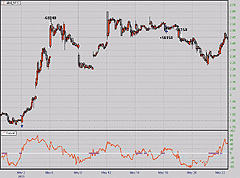FIGURE 11: NEOTICKER, DETECTING BREAKOUTS INTRADAY. The indicator Fvevol  has four plots in total: The first plot is FVE values, the second plot is the EMA of FVE, the third plot is the zero line, and the fourth plot is the alerts, shown as dots along the zero line.

The next one is the formula for the volatility color-coded volume bars. To recreate this formula, create an indicator named volcolor with three parameters: Cintra, Cinter, and Samples (Listing 2). The volcolor indicator has three plots returning the colored volume bars according to the coloring scheme described in the article (Figure 12). The moving average is added as a separate indicator in the chart. Since NeoTicker provides flexible chart indicator alerts, the volume breakout alert can be set as part of the chart alert mechanism.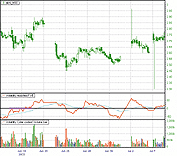FIGURE 12: NEOTICKER, DETECTING BREAKOUTS INTRADAY. The volcolor indicator has three plots returning the colored volume bars according to the coloring scheme described in Markos Katsanos' article. The moving average is added as a separate indicator.

Finally, the FVE strategy can be implemented using a formula indicator/trading system. Create a new indicator named fvest with 10 parameters: Samples, Fveenterl, Fveenteru, MA, Lrperiod, Lrc, UB, LB, and BarToExit. This indicator will plot the current equity of the system (Figure 13). Note the share size of this system varies according to the current available equity; this accounts for the difference in the testing results given in the article. Linear regression slope and tangent constant are used, so in order to change the entry and exit angle, simply use the values BAngle and SAngle. To see detail trades and summary statistics, use the NeoTicker System Performance Viewer (Figure 14).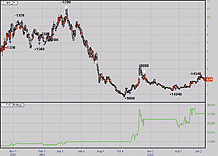FIGURE 13: NEOTICKER, DETECTING BREAKOUTS INTRADAY. The indicator FVEs, which implements the FVE strategy, plots the current equity of the system.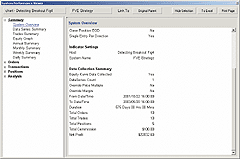FIGURE 14: NEOTICKER, DETECTING BREAKOUTS INTRADAY. Trade  details and summary statistics for the system can be viewed using the NeoTicker System Performance Viewer.

```LISTING 1
Samples := param1;
PERMA   := param2;
CINTRA  := param3;
CINTER  := param4;
xCounter := xCounter+1;
TP     := (h+l+c)/3;
INTRA  := ln(h)-ln(l);
VINTRA := stddev(INTRA, Samples);
INTER  := ln(TP)-ln(TP(1));
VINTER := stddev(INTER, Samples);
CUTOFF := CINTRA*VINTRA+CINTER*VINTER;
MF    := (c-((h+l)/2))+TP-TP(1);
xFVEFactor := if((MF>CUTOFF*c), 1, if(MF<(-1*CUTOFF*c), -1, 0));
volplusminus := v*xFVEFactor;
FVEsum := summation(volplusminus, Samples);
xFVE := (FVEsum/(average(v,Samples)*Samples))*100;
plot1 := average(xFVE, 1);
plot2 := qc_xaverage(xFVE, PERMA);
plot3 := 0;
plot4 := 0;
Condition1 := ((xFVE > -20) and (xFVE < 10));
Condition2 := (xFVE > plot2);
Condition3 := (linslope(xFVE, 20) > 0.5774);
'Plot only if bar number exceed the samples
success1 := if(xCounter > PERMA, 1, 0);
success2 := if(xCounter > PERMA, 1, 0);
success3 := if(xCounter > PERMA, 1, 0);
success4 := if(Condition1 > 0 and Condition2 > 0 and Condition3 > 0, 1, 0);
LISTING 2
CINTRA    := param1;
CINTER    := param2;
Samples   := param3;
TP     := (h+l+c)/3;
INTRA  := ln(h)-ln(l);
VINTRA := stddev(INTRA, Samples);
INTER  := ln(TP)-ln(TP(1));
VINTER := stddev(INTER, Samples);
CUTOFF := CINTRA*VINTRA+CINTER*VINTER;
MF     := (c-(h+l)/2)+TP -TP(1);
plot1 := 0;
plot2 := v;
plot3 := if(MF > (CUTOFF*c), clGreen, if(MF < (-1*CUTOFF*c), clRed, clBlue));
LISTING 3
Samples   := param1;
FVEENTERL := param2;
FVEENTERU := param3;
MA  := param4;
LRPERIOD := param5;
BANGLE := 0.5774;
SANGLE := -0.5774;
LRC := param6;
UB := param7;
LB := param8;
BarToExit := param9;
CINTRA    := 0.1;
CINTER    := 0.1;
myCounter := myCounter+1;
LongCounter := if(OpenPositionLong > 0, LongCounter+1, 0);
TP := (h+l+c)/3;
INTRA  := ln(h)-ln(l);
VINTRA := stddev(INTRA, Samples);
INTER  := ln(TP)-ln(TP(1));
VINTER := stddev(INTER, Samples);
CUTOFF := CINTRA*VINTRA+CINTER*VINTER;
MF := (c -(h+l)/2)+TP-TP(1);
FVEFactor := if(MF > CUTOFF*c, 1, if(MF < -1*CUTOFF*c, -1, 0));
VolPlusMinus := v*FVEFactor;
FVESum := Summation(VolPlusMinus, Samples);
xFVE := (FVESum/(average(v, Samples)*Samples))*100;
Condition1 := ((xFVE > FVEENTERL) and (xFVE < FVEENTERU));
Condition2 := (linslope(xFVE, LRPERIOD) > BANGLE);
Condition3 := xFVE > qc_xaverage(xFVE, MA);
Condition4 := linslope(c, LRC) < UB*linreg(c,LRC,LRC-1)/100 and
linslope(c, LRC) > LB*linreg(c,LRC,LRC-1)/100;
Condition5 := linslope(xFVE, LRPERIOD) < SANGLE;
Condition6 := OpenPositionFlat > 0;
Condition7 := myCounter > 2*Samples;
'Find the number of shares to the nearest of 10
'system can buy with current equity.
ShareNum := int(CurrentEquity/c);
ShareNum := ShareNum - mod(ShareNum, 10);
LongAtMarket(Condition1 > 0 and Condition2 > 0 and Condition3 > 0 and
Condition4 > 0 and Condition6 > 0 and Condition7 > 0, ShareNum);
LongExitAtMarket(Condition5 > 0, OpenPositionAbsSize);
LongExitAtMarket(LongCounter >= BarToExit, OpenPositionAbsSize);
plot1 := CurrentEquity;
success1 := if(Condition7 > 0, 1, 0);```

A downloadable version of this indicator is available from the Yahoo! NeoTicker user group file area at http://groups.yahoo.com/group/neoticker/.

--Kenneth Yuen, TickQuest Inc.
www.tickquest.comNEOTICKER: INDEX OF CHART SENTIMENT

To implement the concept presented in "Index Of Chart Sentiment" by Viktor Likhovidov, first create a formula indicator named CandleCode, with two parameters Period and Offset (Listing 1). This indicator returns one plot. Next, create another formula indicator, chart sentiment index, with four parameters: Period1, Period2, Period BBands, and Offset BBands (Listing 2). This indicator also returns one plot (Figure 15).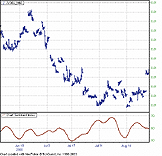FIGURE 15: NEOTICKER, INDEX OF CHART SENTIMENT. The chart sentiment index with four parameters (Period1, Period2, Period BBands, and Offset BBands) returns one plot.
```LISTING 1
myPeriod := param1;
myOffSet := param2;
myCounter := myCounter+1;
xBody  := absvalue(o-c);
Lshd   := if(c>=o, o-l, c-l);
Ushd   := if(c>=o, h-c, h-o);
ThBotB := bbands3.plot2 (0, xBody, myPeriod, myOffSet);
ThTopB := bbands3.plot3 (0, xBody, myPeriod, myOffSet);
ThBotL := bbands3.plot2 (0, Lshd,  myPeriod, myOffSet);
ThTopL := bbands3.plot3 (0, Lshd,  myPeriod, myOffSet);
ThBotU := bbands3.plot2 (0, Ushd,  myPeriod, myOffSet);
ThTopU := bbands3.plot3 (0, Ushd,  myPeriod, myOffSet);
plot1 := if(c=o,1,0)*if(Ushd>=Lshd,64,48)+if(c=o,0,1)*(if(c>o,1,0)*
(if(xBody<=ThBotB,80,0)+if(xBody>ThBotB and xBody<=ThTopB,96,0)+
if(xBody>ThTopB,112,0))+if(c<o,1,0)*(if(xBody<=ThBotB,32,0)+
if(xBody>ThBotB and xBody<=ThTopB,16,0)))+(if(Lshd=0,3,0)+
if(Lshd<ThBotL and Lshd>0,2,0)+
if(Lshd>ThBotL and Lshd<=ThTopL and Lshd>0,1,0))+
(if(Ushd>0 and Ushd<=ThBotU,4,0)+if(Ushd>ThBotU and Ushd<=ThTopU,8,0)+
if(Ushd>ThTopU,12,0));
success1 := if(myCounter>myPeriod, 1, 0);
LISTING 2
Period1 := param1;
Period2 := param2;
BBandPeriod := param3;
BBandOffset := param4;
plot1 := average(average(candlecode(data1, BBandPeriod, BBandOffset), Period1), Period2);```
Downloadable versions of both indicators are available from the Yahoo! NeoTicker user group file area at http://groups.yahoo.com/group/neoticker/.
--Kenneth Yuen, TickQuest Inc.
www.tickquest.com
GO BACKTRADINGSOLUTIONS: DETECTING BREAKOUTS INTRADAY

In "Detecting Breakouts In Intraday Charts," Markos Katsanos presents an extended version of his finite volume elements (FVE) indicator.

The primary new function is a "volatility modified" version of the FVE. This function uses the previous money flow calculation and adds a calculated cutoff coefficient to the equation.

```Name: FVE Money Flow
Short Name: FVEMF
Inputs: Close, High, Low
Formula:
Add (Sub (Close, MP (High, Low)),Change (Typical (Close, High, Low),1))
Name: FVE Cutoff
Short Name: FVECutoff
Inputs: Close, High, Low, Period, Coefficient
Formula:
Mult (Coefficient, Mult (Add (StDev (Sub (Ln (Typical
(Close,High,Low)),Lag (Typical (Close,High,Low),1)),
Period),StDev (Sub (Ln (High),Ln (Low)),Period)),Close))
Name: Finite Volume Elements (Volatility Modified)
Short Name: FVEVM
Inputs: Close, High, Low, Volume, Period, Coefficient
Formula:
Mult (Div (Div (Sum (If (GT (FVEMF (Close, High, Low),
FVECutoff (Close, High, Low, Coefficient, Period)),
Volume, If (LT (FVEMF (Close, High, Low),Negate
(FVECutoff (Close, High, Low, Coefficient, Period))),
Negate (Volume),0)),Period),MA (Volume, Period)),Period),100)```

The output of this function can be colored-coded in the chart in the same way it is in the MetaStock example given in the article. In this case, create three different fields, then use "Change Display Properties of Field..." to change each field to the appropriate color and to use a bar chart.

```Name: FVE Volume Up (Green)
Short Name: FVEVolUp
Inputs: Close, High, Low, Volume, Period, Coefficient
Formula:
If (GT (FVEMF (Close, High, Low),FVECutoff (Close,
High, Low, Coefficient, Period)), Volume,0)
Name: FVE Volume Down (Red)
Short Name: FVEVolDown
Inputs: Close, High, Low, Volume, Period, Coefficient
Formula:
If (LT (FVEMF (Close, High, Low), Negate (FVECutoff
(Close, High, Low, Coefficient, Period))), Volume,0)
Name: FVE Volume Neutral (Blue)
Short Name: FVEVolNeutral
Inputs: Close, High, Low, Volume, Period, Coefficient
Formula:
If (And (LT (FVEMF (Close, High, Low), FVECutoff (Close,
High, Low, Coefficient, Period)),GT (FVEMF (Close, High,
Low), Negate (FVECutoff (Close, High, Low, Coefficient,
Period)))),Volume,0)```

In addition, an entry/exit system was presented for testing trading against the Fve:

```Name: FVE System
Inputs: Close, High, Low, Volume, Period, Coefficient
Enter Long:
1. LT (FVEVM (Close, High, Low, Volume, Period, Coefficient), 10 )
2. GT (FVEVM (Close, High, Low, Volume, Period, Coefficient), -20 )
3. GT (Slope (FVEVM(Close, High, Low, Volume, Period, Coefficient) , 20 ) , 0.58 )
4. GT (FVEVM(Close, High, Low, Volume, Period, Coefficient),
EMA (FVEVM(Close, High, Low, Volume, Period, Coefficient) , 40 ) )
5. LT (Slope(Close, 30), Div (Mult ( Lag ( Close, 30), 0.6, 100) )
6. GT (Slope(Close, 30), Negate (Div (Mult ( Lag ( Close, 30), 0.3, 100) ))
Exit Long:
1. LT (Slope (FVEVM(Close, High, Low, Volume, Period, Coefficient) , 20 ) , 0.58 )```

These functions are available in a function file that can be downloaded from the TradingSolutions website in the Solution Library section.

As with many indicators, functions such as the FVE can make good inputs to neural network predictions. If used directly, you will want to set the preprocessing to "none," since the value stays within a specific range, or set it to "change" if the momentum of the indicator is desired.

--Gary Geniesse, NeuroDimension, Inc.
800 634-3327, 352 377-5144
GO BACKINVESTOR/RT: DETECTING BREAKOUTS INTRADAY

The FVE as described by Markos Katsanos in "Detecting Breakouts In Intraday Charts" can be implemented in Investor/RT using a custom indicator with the following syntax:

`SUM((CI / VMA)/22, 22) * 100`

where CI is in turn an embedded custom indicator with the following syntax:

```(((CL - MED) + (MID - MID.1)) > ( 0.003 * CL)) * VO +
(((CL - MED) + (MID - MID.1)) < (-0.003 * CL)) * (VO * -1)```

The finite volume elements (FVE) indicator can be seen in the lower pane of the chart in Figure 16.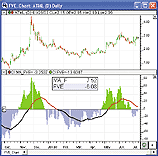FIGURE 16: INVESTOR/RT, FINITE VOLUME ELEMENTS. This Investor/RT daily candlestick chart of ATML shows the FVE indicator in the lower pane, overlaid with a 40-period exponential moving average of FVE.

In this case, we have set up the FVE with a period of 22 and a cutoff coefficient of 0.30%, according to the table in Figure 1 of Katsanos' article. These two numbers should be adjusted according to this table based on the periodicity of the underlying chart, scan, or backtest.

In Figure 16, a 40-period exponential moving average of FVE is also present. This can be achieved by creating the following custom indicator:

`MA(CI)`

in which CI is set up as the FVE custom indicator created above, and MA is set up as a 40-period exponential moving average.

Any Investor/RT user can quickly import this chart by visiting the web page: http://www.linnsoft.com/charts/

By importing the chart, the user will also import the custom indicators required to create the FVE, and the moving average of the FVE. The size, colors, symbol, indicators, scaling properties, and all other characteristics of the chart will also be reflected on the imported chart.

http://www.linnsoft.com/tour/customIndicator.htm
http://www.linnsoft.com/tour/techind/movAvg.htm
http://www.linnsoft.com/tour/techind/volume.htm

800-546-6842, info@linnsoft.com
www.linnsoft.comINVESTOR/RT: CHART SENTIMENT INDEX

Investor/RT's implementation of the CandleCode indicator can be seen in the lower pane of Figure 17. The blue line seen in the lower pane represents the double-smoothed CandleCode indicator (CC). This double-smoothed CC is what is described as the index of chart sentiment in this month's article by Viktor Likhovidov. The preferences used for the CC in Figure 17 can be seen in Figure 18.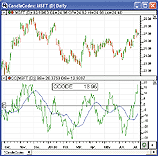FIGURE 17: Investor/RT, Index of Chart Sentiment. This Investor/RT daily candlestick chart of MSFT shows the CandleCode (CC) indicator in the lower pane, with the green line representing the single smoothing of CC, and the blue line representing a double smoothing of CC.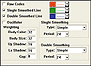FIGURE 18: Investor/RT. Here are the preferences used for the CandleCode indicator seen charted in Figure 17.

The Investor/RT CandleCode (CC) indicator allows the user to provide weights (importance factors) to each of the following candle features: body color, body size, upper shadow, lower shadow, and gap. The raw CC values can be single and/or double-smoothed. An oscillator may be drawn representing the difference between the single-and double-smoothed values.

800-546-6842, info@linnsoft.com
www.linnsoft.comFINANCIAL DATA CALCULATOR: DETECTING BREAKOUTS INTRADAY

"Detecting Breakouts In Intraday Charts" by Markos Katsanos in this issue introduces the modified FVE, using volatility for determining the cutoff. This indicator can be programmed as a macro in Financial Data Calculator (FDC) as follows.

Open the macro wizard, choose "New macro," and enter the following code into the definition window:

```H: hi #R
L: lo #R
C: cl #R
V: vol #R
T: typical #R
M: midrange #R
numbars: #L
interv: numbars movstdv change log T
intrav: numbars movstdv (log H) - (log L)
threshold: 0.1*(interv+intrav)*C
mf: (C - M)-change T
sign: (mf > threshold) - (mf < threshold)
signedv: sign * V
100*(numbars movsum signedv)/(numbars movsum V)```

Save the macro as the name "fve." The intermediate abbreviations to the left of the colons are there mostly to aid readability. The final line defines the FVE macro (note that we have taken advantage of the fact that t times a t-bar moving average is just a t-bar moving sum).

This macro allows inputs for the number of bars and the target dataset. For example, if you entered the line "22 Fve Ibm" in the FDC command window, the output would be the 22-bar FVE for the dataset Ibm.

The FVE macro takes advantage of two other simple macros that are resident in FDC: The macro "Typical" computes one-third of the high+low+close of a dataset, and "Midrange" returns the (high+low)/2 of a dataset.

Katsanos uses this indicator to produce the buy entry signal in a trade simulation. The four conditions for entry can be placed directly in one or more of the "buy entry conditions" for a new trade in the trade wizard; but in this case it makes the trade structure more obvious if a macro is first built for the (quite complex) buy entry.

We create the macro as follows:

```f: 22 fve #R
cond1: (-20 < f) and (10 > f)
cond2: (arctan 20 movslope f) > .524
cond3: f > 40 expave f
a: (30 movslope cl #R) < .0051* 30 movtrend cl #R
b: (30 movslope cl #R) > (-.0033)* 30 movtrend cl #R
cond1 and cond2 and cond3 and a and b```

This macro is then saved under the name "fvebuy." In the macro, f is the 22-bar FVE of the target dataset. Condition 1 is that this FVE be between -20 and 10. Condition 2 states that the angle of the 20-day moving regression line be > 30 degrees (expressed as 0.524 radians). Condition 3 states that the FVE exceeds its 40-day exponential moving average. Finally, conditions a and b make up condition 4 of the article.

In Financial Data Calculator, the function movtrend returns the current value of the moving regression line, and movslope returns the slope of that line. The value of the regression line k days ago is found by subtracting k times the slope from the trend value. When the formula for condition 4 in the article is expressed this way and simplified, a and b result.

We now form a trade function. Open the trade wizard, choose "New trade" and in the first buy entry screen fill in the information shown in Figure 19. Then choose the first buy exit screen and fill it in as shown in Figure 20. This says that we should exit if the angle of the 20-day moving average of the 22-bar FVE is -20 degrees (-0.349 radians) or less. The author suggests that a time stop of 50 bars could also be useful. This could be added by putting in the corresponding line of the second exit method screen the line "#numbars > 50".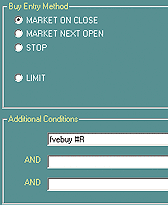FIGURE 19: FINANCIAL DATA CALCULATOR, MODIFIED FVE. To create the trade function for the volume-modified FVE, open the trade wizard, choose "New trade," and in the first buy entry screen, fill in the information shown here.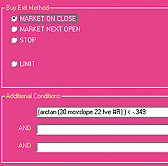FIGURE 20: FINANCIAL DATA CALCULATOR, MODIFIED FVE. Next, choose the first buy exit screen and fill it in as shown here.

--Robert Busby
Futures Software Associates, Inc.
856 857-9088
www.financialdatacalculator.com